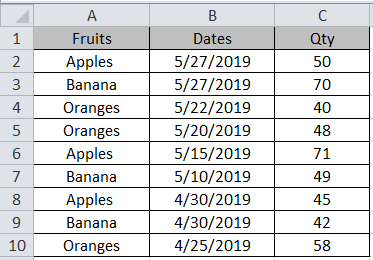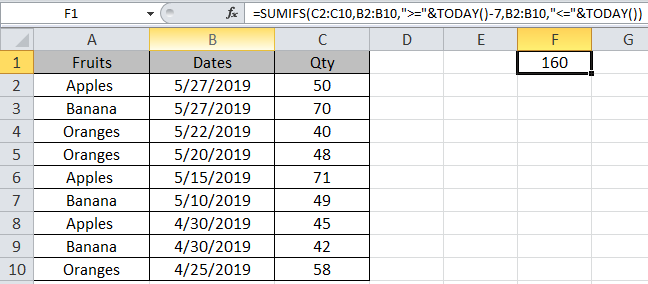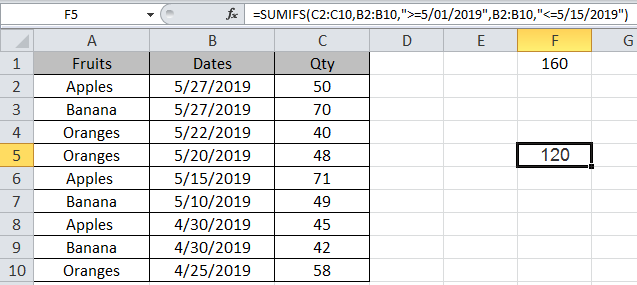# How to use the SUMIFS with Dates in Excel

In this article, we will learn how to use the SUMIFS function with dates in Excel 2016. The question here comes is what is SUMIFS function? What it does and how you will use it for your data set.

We will learn it all step by step.

SUMIFS function is used where we need to find the sum under some given conditions. Under many circumstances, SUMIFS is used to reduce the user’s input.
Syntax:

=SUMIFS(sum_range, criteria_range1, criteria1, [criteria_range2, criteria2], …)

Let’s take an example to get thisHere is a list of Fruits and its quantity with dates.

We will do this in two cases:

1st case
We need to find the SUM of quantity of fruits 7 days from Today.
Today is 5/27/2019.

Formula in F1 cell

=SUMIFS(C2:C10,B2:B10,">="&TODAY()-7,B2:B10,"<="&TODAY())

Explanation:
SUM of quantity is in range C2:C10
Criteria is within last 7 days. So 1st criteria would be Dates lesser than today and 2nd criteria would be Dates greater than 7 days from Today.
“>=”&Today()-7 Dates greater than 7 days from now.
“<=”&Today() Dates less than TodayThe sum of 50+70+40 = 160

2nd Case
We need to find the sum of Qty of fruits between 2 given dates.

Formula in F5 cell:
=SUMIFS(C2:C10, B2:B10, “>=5/01/2019", B2:B10, “<=5/15/2019")SUM of quantity is in range C2:C10
Criteria is within last 7 days. So 1st criteria would be Dates lesser than today and 2nd criteria would be Dates greater than 7 days from Today.
“>=”& 5/01/2019 Dates after 5/01/2019.
“<=”& 5/15/2019 Dates before 5/15/2019The Sum of 71+49 = 120
As you can see the Sum in the required cell.

Hope you understood how to use SUMIFS function with dates in Excel 2016 with the above examples. You can perform these tasks in Excel 2013 and 2010. Find more articles on Mathematical formulation and logic test functions. Please state any unresolved query you have in the comment box below. We will help you.

Popular Articles:

50 Excel Shortcuts to Increase Your Productivity

How to use the VLOOKUP Function in Excel

How to use the COUNTIF function in Excel

How to use the SUMIF Function in Excel

Terms and Conditions of use

The applications/code on this site are distributed as is and without warranties or liability. In no event shall the owner of the copyrights, or the authors of the applications/code be liable for any loss of profit, any problems or any damage resulting from the use or evaluation of the applications/code.